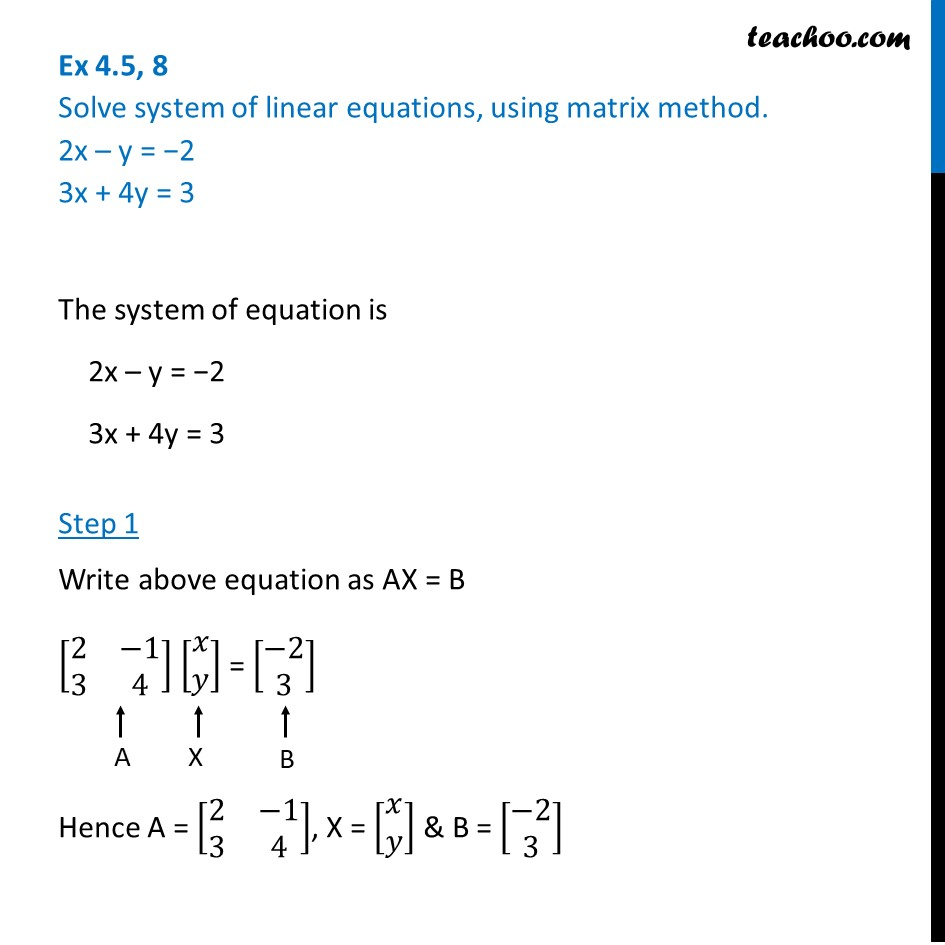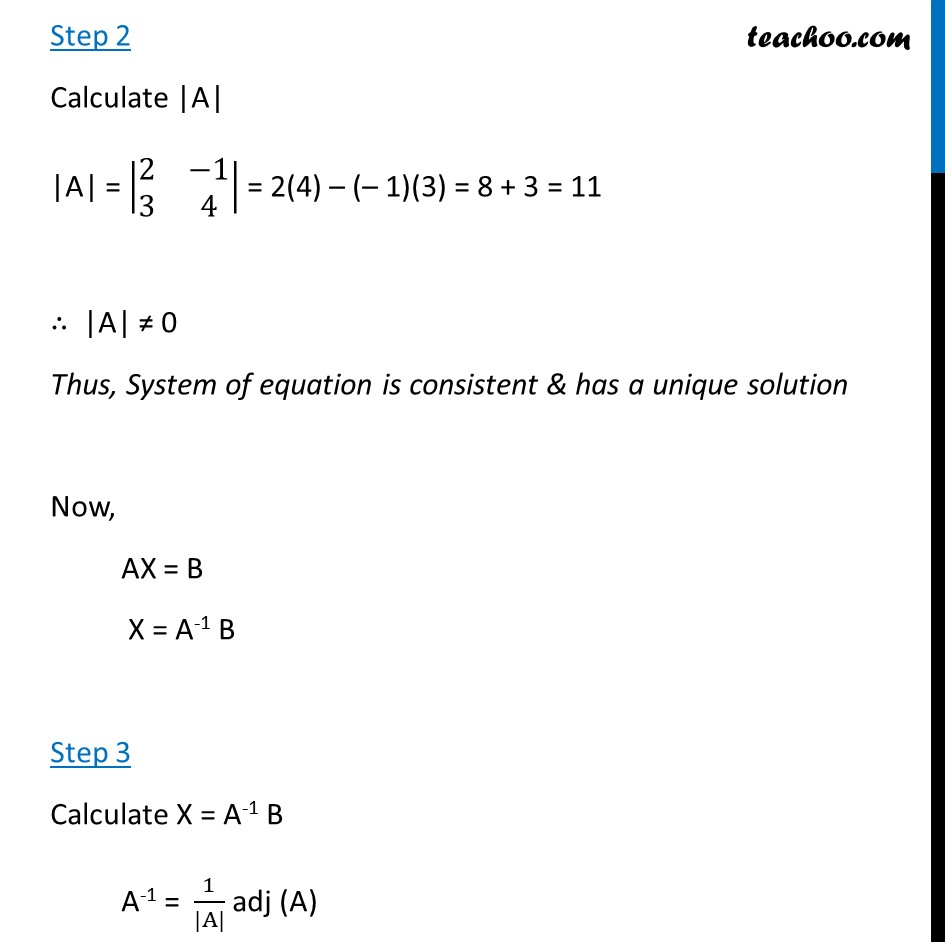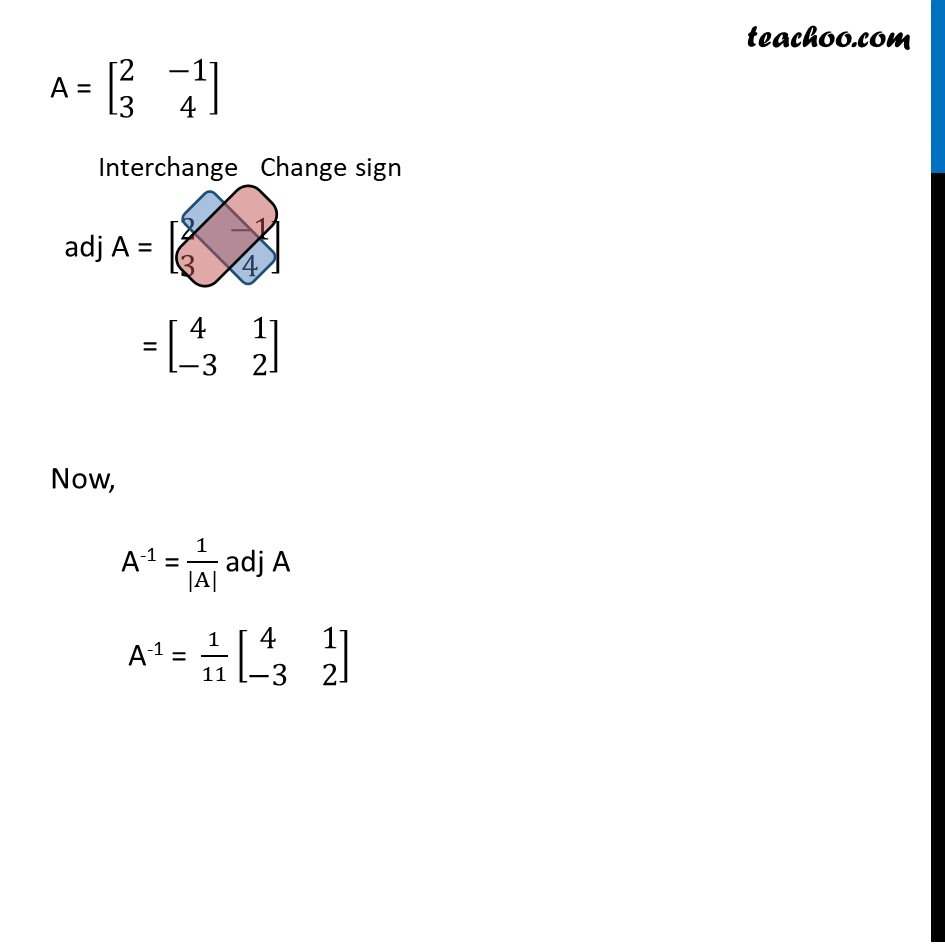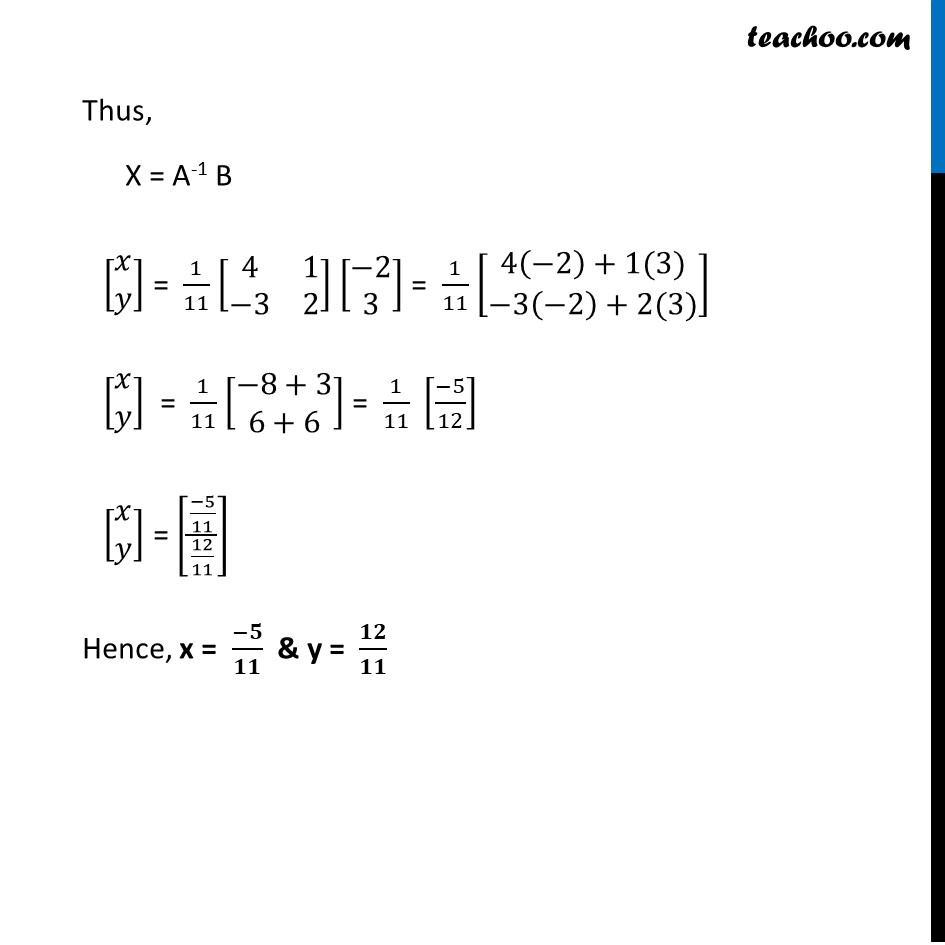Ex 4.5

Chapter 4 Class 12 Determinants
Serial order wiseLearn in your speed, with individual attention - Teachoo Maths 1-on-1 Class

### Transcript

Ex 4.5, 8 Solve system of linear equations, using matrix method. 2x – y = −2 3x + 4y = 3 The system of equation is 2x – y = −2 3x + 4y = 3 Step 1 Write above equation as AX = B [■8(2&−1@3&4)] [■8(𝑥@𝑦)] = [■8(−2@3)] Hence A = [■8(2&−1@3&4)], X = [■8(𝑥@𝑦)] & B = [■8(−2@3)] Step 2 Calculate |A| |A| = |■8(2&−1@3&4)| = 2(4) – (– 1)(3) = 8 + 3 = 11 ∴ |A| ≠ 0 Thus, System of equation is consistent & has a unique solution Now, AX = B X = A-1 B Step 3 Calculate X = A-1 B A-1 = 1/(|A|) adj (A) Interchange Thus, X = A-1 B [■8(𝑥@𝑦)] = 1/11 [■8(4&1@−3&2)] [■8(−2@3)] = 1/11 [■8(4(−2)+1(3)@−3(−2)+2(3))] [■8(𝑥@𝑦)] = 1/11 [■8(−8+3@6+6)] = 1/11 [(−5)/12] [■8(𝑥@𝑦)] = [((−5)/11)/(12/11)] Hence, x = (−𝟓)/𝟏𝟏 & y = 𝟏𝟐/𝟏𝟏